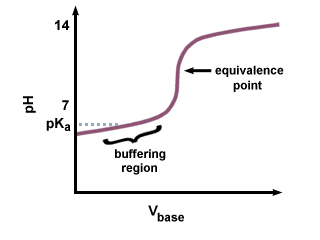# The Titration Experiment

Titration is a general class of experiment where a known property of one solution is used to infer an unknown property of another solution. In acid-base chemistry, we often use titration to determine the pH of a certain solution.

A setup for the titration of an acid with a base is shown in :

We use this instrumentation to calculate the amount of unknown acid in the receiving flask by measuring the amount of base, or titrant, it takes to neutralize the acid. There are two major ways to know when the solution has been neutralized. The first uses a pH meter in the receiving flask adding base slowly until the pH reads exactly 7. The second method uses an indicator. An indicator is an acid or base whose conjugate acid or conjugate base has a color different from that of the original compound. The color changes when the solution contains a 1:1 mixture of the differently colored forms of the indicator. As you know from the Henderson-Hasselbalch equation, the pH equals the pK a of the indicator at the endpoint of the indicator. Since we know the pH of the solution and the volume of titrant added, we can then deduce how much base was needed to neutralize the unknown sample.

### Titration Curves

A titration curve is drawn by plotting data attained during a titration, titrant volume on the x-axis and pH on the y-axis. The titration curve serves to profile the unknown solution. In the shape of the curve lies much chemistry and an interesting summary of what we have learned so far about acids and bases.

The titration of a strong acid with a strong base produces the following titration curve:

Note the sharp transition region near the equivalence point on the . Also remember that the equivalence point for a strong acid-strong base titration curve is exactly 7 because the salt produced does not undergo any hydrolysis reactions.

However, if a strong base is used to titrate a weak acid, the pH at the equivalence point will not be 7. There is a lag in reaching the equivalence point, as some of the weak acid is converted to its conjugate base. You should recognize the pair of a weak acid and its conjugate base as a buffer. In , we see the resultant lag that precedes the equivalence point, called the buffering region. In the buffering region, it takes a large amount of NaOH to produce a small change in the pH of the receiving solution.The titration of a base with an acid produces a flipped-over version of the titration curve of an acid with a base. pH is decreased upon addition of the acid.

Note that the pH of a solution at the equivalence point has nothing to do with the volume of titrant necessary to reach the equivalence point; it is a property inherent to the composition of the solution. The pH at the equivalence point is calculated in the same manner used to calculate the pH of weak base solutions in Calculating pH's.

When polyprotic acids are titrated with strong bases, there are multiple equivalence points. The titration curve of a polyprotic acid shows an equivalence point for the each protonation:Because the conjugate base is basic, the pH will be greater than 7 at the equivalence point. You will need to calculate the pH using the Henderson-Hasselbalch equation, and inputting the pK b and concentration of the conjugate base of the weak acid.

The titration curve shown above is for a diprotic acid such as H2SO4 and is not unlike two stacked . For a diprotic acid, there are two buffering regions and two equivalence points. This proves the earlier assertion that polyprotic acids lose their protons in a stepwise manner.